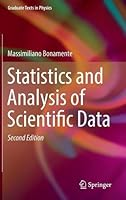# Statistics and Analysis of Scientific Data, 2nd Edition0 Reviews
2016-11-09
318 pages

## Book Description

The revised second edition of this textbook provides the reader with a solid foundation in probability and statistics as applied to the sciences, and related fields. It covers a broad range of numerical and analytical methods that are essential for the correct analysis of scientific data, including probability theory, distribution functions of statistics, fits to two-dimensional data and parameter estimation, Monte Carlo methods and Markov chains.

Features new to this edition include:

• a discussion of statistical techniques employed in science, such as multiple regression analysis of multivariate datasets.
• a new chapter on the various measures of the mean including logarithmic averages.
• new chapters on systematic errors and intrinsic scatter, and on the fitting of data with bivariate errors.
• a new case study and additional worked examples.
• mathematical derivations and theoretical background material have been appropriately marked, to improve the readability of the text.
• end-of-chapter summary boxes, for easy reference.

As in the first edition, the main pedagogical method is a theory-then-application approach, where emphasis is placed first on a sound understanding of the underlying theory of a topic, which becomes the basis for an efficient and practical application of the material. The level is appropriate for undergraduates and beginning graduate students, and as a reference for the experienced researcher. Basic is used in some of the derivations, and no previous background in probability and statistics is required. The book includes many numerical tables of data, as well as exercises and examples to aid the readers' understanding of the topic.

Chapter 1 Theory of Probability
Chapter 2 Random Variables and Their Distributions
Chapter 3 Three Fundamental Distributions: Binomial, Gaussian, and Poisson
Chapter 4 Functions of Random Variables and Error Propagation
Chapter 5 Maximum Likelihood and Other Methods to Estimate Variables
Chapter 6 Mean, Median, and Average Values of Variables
Chapter 7 Hypothesis and Statistics
Chapter 8 Maximum Likelihood Methods for Two-Variable Datasets
Chapter 9 Multi-Variable Regression
Chapter 10 Goodness of Fit and Parameter Uncertainty
Chapter 11 Systematic Errors and Intrinsic Scatter
Chapter 12 Fitting Two-Variable Datasets with Bivariate Errors
Chapter 13 Model Comparison
Chapter 14 Monte Carlo Methods
Chapter 15 Introduction to Markov Chains
Chapter 16 Monte Carlo Markov Chains
Appendix: Numerical Tables

## Book Details

• Title: Statistics and Analysis of Scientific Data, 2nd Edition
• Author:
• Length: 318 pages
• Edition: 2nd ed. 2017
• Language: English
• Publisher:
• Publication Date: 2016-11-09
• ISBN-10: 1493965700
• ISBN-13: 9781493965700